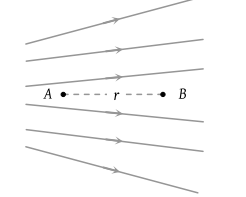You’ve reached the end of your free Videos limit.
#7 | Electric Field Lines & Intensity
(Physics) > Electric Charges and Fields
Unable to watch the video, please try another server
Related Practice Questions :

You are traveling in a car during a thunderstorm. In order to protect yourself from lightning, you would prefer to

(1) Touch the nearest electric pole

(2) Take shelter under a tree

(3) Get out and crouch on the ground

(4) Remain in the car

High Yielding Test Series + Question Bank - NEET 2020

Difficulty Level:

The electrostatic field due to a charged conductor just outside the conductor is

1. zero and parallel to the surface at every point inside the conductor

2. zero and is normal to the surface at every point inside the conductor

3. parallel to the surface at every point and zero inside the conductor

4. normal to the surface at every point and zero inside the conductor

High Yielding Test Series + Question Bank - NEET 2020

Difficulty Level:

In the absence of other conductors, the surface charge density

(1) Is proportional to the charge on the conductor and its surface area

(2) Inversely proportional to the charge and directly proportional to the surface area

(3) Directly proportional to the charge and inversely proportional to the surface area

(4) Inversely proportional to the charge and the surface area

High Yielding Test Series + Question Bank - NEET 2020

Difficulty Level:

Two equally charged, identical metal spheres A and B repel each other with a force 'F'. The spheres are kept fixed with a distance 'r' between them. A third identical, but uncharged sphere C is brought in contact with A and then placed at the mid-point of the line joining A and B. The magnitude of the net electric force on C is

(1) F

(2) 3F/4

(3) F/2

(4) F/4

High Yielding Test Series + Question Bank - NEET 2020

Difficulty Level:

Figure shows the electric lines of force emerging from a charged body. If the electric field at A and B are EA and EB respectively and if the displacement between A and B is r then(1) EA > EB

(2) EA < EB

(3) ${E}_{A}=\frac{{E}_{B}}{r}$

(4) ${E}_{A}=\frac{{E}_{B}}{{r}^{2}}$